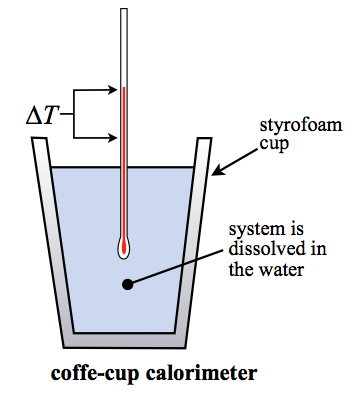# Calorimetry Study Guide

What Is a Calorimeter?

A calorimeter is a device used to measure the amount of heat released or absorbed during a chemical or physical reaction. The term “calorimeter” comes from the Latin word “calor,” meaning heat.

Calorimeters are often used in chemistry and physics experiments to determine the heat released or absorbed by a reaction or process. This information can be used to calculate the enthalpy (heat content) of a substance or reaction, as well as other thermodynamic properties.

### PRINCIPLE OF CALORIMETRY

The law of conservation of energy is the calorimeter’s primary concept. One body’s heat loss is balanced by the heat acquired by another. The principle of calorimetry is widely used in thermochemistry to calculate enthalpy, stability, and heat capacity, among other things.

The formula of calorimetry is as follows –

𝑄𝑠𝑢𝑏 =𝑚×𝐶×Δ𝑇

Where 𝑄𝑠𝑢𝑏 = the heat energy of a body

m= mass of the body

C= specific heat

And Δ𝑇 is the temperature difference

## USES OF CALORIMETRY

• The energy of matter has a significant impact on its natural occurrence, reactivity, and other properties.
• If we can figure out how they are related, we can use the energy measurements.
• Some uses of calorimetrycan be to forecast natural occurrence, reactivity, and physical attributes.
• Understanding a substance’s thermodynamic quantities will always lead to answers about its structure and other properties.

## TYPES OF CALORIMETERSA calorimeter is a device that measures heat and is used in calorimetry. There are different types of calorimeters that are used; some of them are:

• Constant pressure calorimeter
• Reaction calorimeters
• Differential scanning calorimeter
• Bomb calorimeters

# SUMMARY

• Calorimetry is a branch of science concerned with determining a body’s state in terms of thermal features.
• The law of conservation of energy is the calorimeter’s primary concept.
• The formula of calorimetry is 𝑄𝑠𝑢𝑏 =𝑚×𝐶×Δ𝑇

## FAQs

Q. What is the main requirement for a calorimeter?

__Answer: __ A metallic vessel ( a good conductor of electricity ) is the main requirement of a calorimeter.

Q. Why is water used in calorimetry?

__Answer: __Water is used in calorimetry because it has a high specific heat capacity.

Q. What is a limitation of the calorimetry method?

__Answer: __ Losing heat to the environment and uneven heating is the limitation of the calorimetry.

We hope you enjoyed studying this lesson and learned something cool about Calorimetry! Join our Discord community to get any questions you may have answered and to engage with other students just like you! Don’t forget to download our App to experience our fun VR classrooms – we promise, it makes studying much more fun! 😎

]]>